# Predicting House prices using the Ames, Iowa house dataset

## Predicting House prices using the Ames, Iowa house dataset

Updated: Sep 20, 2019

##Introduction

Kaggle offers an ongoing competition that allows students of Machine Learning to build a model to predict house prices using a dataset of 80 features. The dataset includes many features one would consider relevant in buying a house, such as lot area, square feet of living area, number of bedrooms, number of bathrooms, etc.

The dataset is broken up into a training dataset and a test dataset. The training dataset includes 1460 observations, and includes the sale price of the house. The test dataset includes 1459 observations and does not include the sale price of the home.

The goal of the project is to develop a predictive model to predict the value of a house using the features provided.

## Data Cleaning

A major portion of the work was preparing the data for modeling. There were a large amount of Nas in both the training and test set. When looking at the data, it became clear that many of the Nas were not missing values at all, but could be filled in using basic logic. For example, there were many features related to the basement that had Nas. However, it turns out that many of the houses in these observations did not have a basement, which was easily verified by looking the basement square feet column. Thus, I filled in the Nas for these observations with "None".

The same idea applied to many of the other columns, such as the garage columns, and pool columns. After the initial cleaning applying basic logic, the number of Nas had been reduced substantially.

## Data Analysis

Before beginning the modelling, I performed a little bit of data analysis. I avoided doing too much, since it is not clear what kind of inferences can be drawn from this kind of analysis. For example, looking at correlations between the features and the sale price may show which features are strongly correlated with the sale price, but does not control for the value of the other variables. However, when interpreting the results of linear regression, for example, one holds the values of all other variables constant, and interprets the coefficient of the feature that is not held constant as the slope. So, relying on correlations where the other variables are not held constant can be misleading.

I did look at the distribution of the sale price. From the histogram below, we see that it has a long tail with many high prices.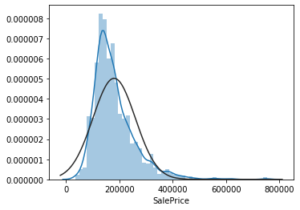Since the scoring metric is root mean square log error, which has the effect of minimizing the differences in errors between high and low valued houses, I decided to log transform the sales price. The histogram of the log transformed sale price is shown below.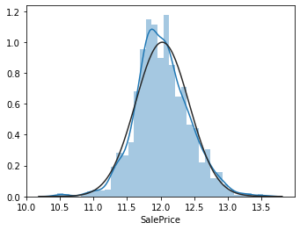## Modeling

### Test Harness

The first step in the modeling process was to come up with a test harness that could be used to test multiple models. I decided to use an initial train-test split of the training data, followed by cross validating each model on the training data before applying the best results to the held out test data.

I used a train-test split of 80/20. I also used scikit learn's Pipeline class to construct a pipeline for cross validation. The advantage of using a pipeline is that data leakage is prevented, since the pipeline automatically takes care of this through all the steps done. The pipeline consisted of splitting the features into numerical and categorical features. For the numerical features, I used median imputation to fill in the missing values, followed by applying a standard scaler transformation. For the categorical features, I used the most frequent imputation strategy followed by one hot encoding the features.

After the data was fed through the preprocessing pipeline, it was ready to be cross validated with the appropriate estimator. I go through each of the estimators below.

### Lasso

I first tried fitting a Lasso model to the data. I tuned the hyperparameter alpha using the range .0005 to 10. The results of the mean square error and standard deviation for each value of alpha are shown below:

 alpha mean square error std dev of error 10 .391 .0094 1 .391 .0094 .1 .207 .005 .001 .112 .003 .0005 .111 .0026

From the data, we see that the optimal hyperparameter is .0005, leading to a cross validated error of .11. When applied to the held out test set, the error was .116. The graph below shows the top features selected using the Lasso: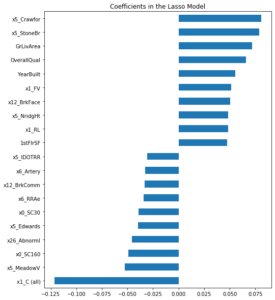### Ridge

I next tried fitting a Ridge model to the data. I again tuned the hyperparameter alpha using the range .0005 to 10. The results of the mean square error and standard deviation for each value of alpha are shown below:

 alpha mean square error std dev 10 .115 .0027 1 .117 .0023 .1 .122 .0023 .001 .125 .0021 .0005 .125 .0021

From the data, we see that the optimal hyperparameter is 10, leading to a cross validated error of .114. When applied to the held out test set, the error was .118. The graph below shows the top features selected using the Ridge: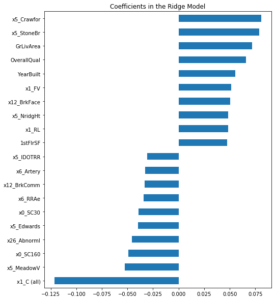### ElasticNet

I next tried fitting an ElasticNet model to the data. There are two hyperparameters for ElasticNet, L1 ratio and alpha. I again tuned the hyperparameter alpha using the range .0005 to 10. I used the range .1 to 1 for the hyperparameter L1 ratio.

From the data, we see that the optimal hyperparameter is  alpha = .001 and l1_ratio = .7, leading to a cross validated error of .114. When applied to the held out test set, the error was .118. The graph below shows the top features selected using ElasticNet: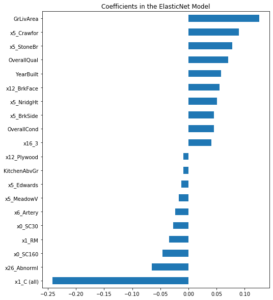### Random Forest

I decided to try a few nonlinear models as well. The first one I tried was Random Forest. The hyperparameters are n_estimators and max_features. I used a range of 100 to 200 for n_estimators, 25 to 75 for max_features, and  2-6 for min_samples_split. The optimal hyperparameters were max_features = 75, n_estimators = 200, and min_samples_split = 4. The test MSE was .134.

### XGBoost

The last model I tried was XGBoost. I tried the hyperparameter ranges of 100 - 500 for n_estimators and max_depth range of 1-3. The optimal hyperparameter from gridsearch were n_estimators = 500 and max_depth - 2. The test set performance was .112

## Conclusion

All of the models I tried performed similarly. The best performance was from XGBoost, which yielded a test error of .112. Future work can include greater feature engineering, and using more ensembling techniques to improve predictions.

### Rohit Parthasarathy

View all articles

Topics from this blog: Machine Learning Student Works

## Interested in becoming a Data Scientist?

Get Customized Course Recommendations In Under a Minute